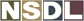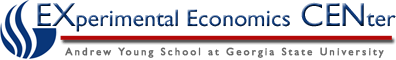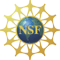Students / Subjects# Interest Rates

There are two different interest rates to consider when looking at inflation.  The first is the nominal interest rate which is the rate that the bank pays.  The real interest rate is the increase in your purchasing power.  The relationship between these two rates and inflation can be noted as follows: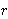=-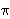Where:= the real interest rate= the nominal interest rate= the rate of inflation

The Fisher Equation is obtained by rearranging this equation to make:=+Irving Fisher developed the equation in this way to represent that the nominal interest rate is based on two things: the inflation rate changing or the real interest rate changing.  Basically, this is saying that a one percent increase in the rate of inflation will cause a corresponding one percent increase in the nominal interest rate.